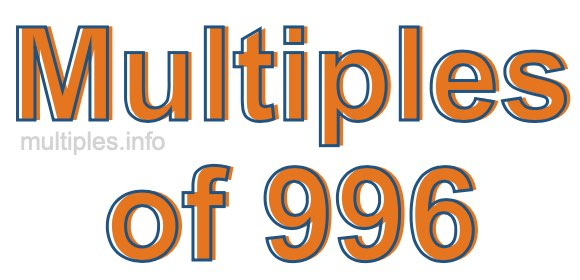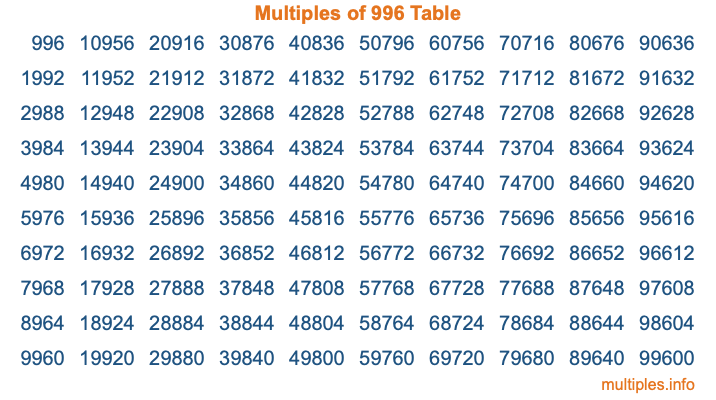Multiples of 996Welcome to the Multiples of 996 page. Here we will first teach you everything you will ever need to know about the multiples of 996, and then give you a study guide summary of everything we taught you to make sure you remember it all. Use this page to look up facts and learn information about the multiples of 996. This page will make you a multiples of nine hundred ninety-six expert!

Definition of Multiples of 996
Multiples of 996 are all the numbers that when divided by 996 equal an integer. Each of the multiples of 996 are called a multiple. A multiple of 996 is created by multiplying 996 by an integer.

Therefore, to create a list of multiples of 996, you start with 1 multiplied by 996, then 2 multiplied by 996, then 3 multiplied by 996, and so on for as long as you want. Thus, the list of the first five multiples of 996 is 996, 1992, 2988, 3984, and 4980. To see a larger list of multiples of 996, see the printable image of Multiples of 996 further down on this page. We also have a category where you can choose any nth multiple of 996.

Multiples of 996 Checker
The Multiples of 996 Checker below checks to see if any number of your choice is a multiple of 996. In other words, it checks to see if there is any number (integer) that when multiplied by 996 will equal your number. To do that, we divide your number by 996. If the the quotient is an integer, then your number is a multiple of 996.

Is  a multiple of 996?

Least Common Multiple of 996 and ...
A Least Common Multiple (LCM) is the lowest multiple that two or more numbers have in common. This is also called the smallest common multiple or lowest common multiple and is useful to know when you are adding our subtracting fractions. Enter one or more numbers below (996 is already entered) to find the LCM.

Check out our LCM Calculator if you need more details about the Least Common Multiple or if you need the LCM for different numbers for adding and subtraction fractions.

nth Multiple of 996
As we stated above, 996 is the first multiple of 996, 1992 is the second multiple of 996, 2988 is the third multiple of 996, and so on. Enter a number below to find the nth multiple of 996.

th multiple of 996

Multiples of 996 vs Factors of 996
996 is a multiple of 996 and a factor of 996, but that is where the similarities end. All postive multiples of 996 are 996 or greater than 996. All positive factors of 996 are 996 or less than 996.

Below is the beginning list of multiples of 996 and the factors of 996 so you can compare:

Multiples of 996: 996, 1992, 2988, 3984, 4980, etc.

Factors of 996: 1, 2, 3, 4, 6, 12, 83, 166, 249, 332, 498, 996

As you can see, the multiples of 996 are all the numbers that you can divide by 996 to get a whole number. The factors of 996, on the other hand, are all the whole numbers that you can multiply by another whole number to get 996.

It's also interesting to note that if a number (x) is a factor of 996, then 996 will also be a multiple of that number (x).

Multiples of 996 vs Divisors of 996
The divisors of 996 are all the integers that 996 can be divided by evenly. Below is a list of the divisors of 996.

Divisors of 996: 1, 2, 3, 4, 6, 12, 83, 166, 249, 332, 498, 996

The interesting thing to note here is that if you take any multiple of 996 and divide it by a divisor of 996, you will see that the quotient is an integer.

Multiples of 996 Table
Below is an image of the first 100 multiples of 996 in a table. The table is in chronological order, column by column. The first column has the first ten multiples of 996, the second column has the next ten multiples of 996, and so on.The Multiples of 996 Table is also referred to as the 996 Times Table or Times Table of 996. You are welcome to print out our table for your studies.

Negative Multiples of 996
Although not often discussed or needed in math, it is worth mentioning that you can make a list of negative multiples of 996 by multiplying 996 by -1, then by -2, then by -3, and so on, to get the following list of negative multiples of 996:

-996, -1992, -2988, -3984, -4980, etc.

Multiples of 996 Summary
Below is a summary of important Multiples of 996 facts that we have discussed on this page. To retain the knowledge on this page, we recommend that you read through the summary and explain to yourself or a study partner why they hold true.

There are an infinite number of multiples of 996.

A multiple of 996 divided by 996 will equal a whole number.

996 divided by a factor of 996 equals a divisor of 996.

The nth multiple of 996 is n times 996.

The largest factor of 996 is equal to the first positive multiple of 996.

996 is a multiple of every factor of 996.

996 is a multiple of 996.

A multiple of 996 divided by a divisor of 996 equals an integer.

996 divided by a divisor of 996 equals a factor of 996.

Any integer times 996 will equal a multiple of 996.

Multiples of a Number
Here you can get the multiples of another number, all with the same attention to detail as we did for multiples of 996 on this page.

Multiples of
Multiples of 997
Did you find our page about multiples of nine hundred ninety-six educational? Do you want more knowledge? Check out the multiples of the next number on our list!